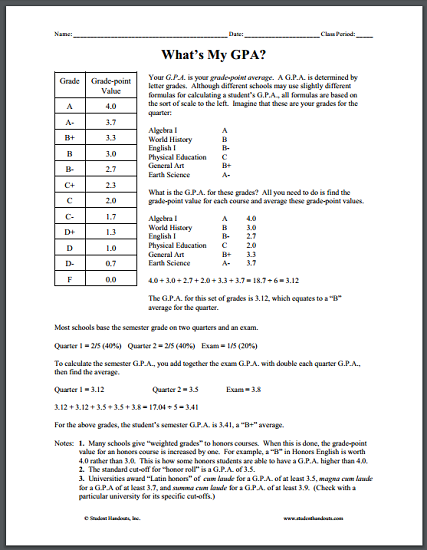How to Calculate Your G.P.A. | Student Handouts
 Share the learning joy!
Free Printable Handout for Teachers & Students - Scroll Down to Print (PDF) - Grades - Sub Folder

Although different schools may use slightly different formulas for calculating a student’s G.P.A., all formulas are based on the sort of scale to the left [see worksheet]. Imagine that these are your grades for the quarter: Algebra I-A; World History-B; English I-B; Physical Education-C; General Art-B+; Earth Science-A.

What is the G.P.A. for these grades? All you need to do is find the grade-point value for each course and average these grade-point values.

Algebra I-A 4.0; World History-B 3.0; English I-B- 2.7; Physical Education-C 2.0; General Art-B+ 3.3; Earth Science-A- 3.7; then tally them up and find the average: 4.0 + 3.0 + 2.7 + 2.0 + 3.3 + 3.7 = 18.7 ÷ 6 = 3.12. The G.P.A. for this set of grades is 3.12, which equates to a “B” average for the quarter.